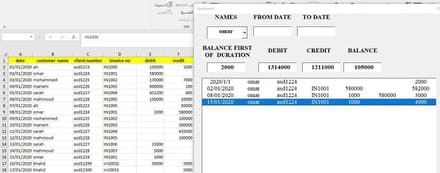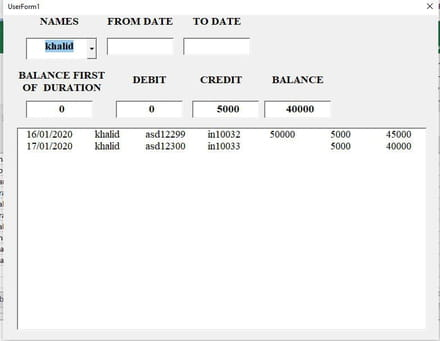# Fixing populating values into textboxes based on showing data in listbox

Solved/Closed
abdelfatah_0230 Posts 73 Registration date Thursday July 18, 2019 Status Member Last seen July 23, 2022 - Dec 2, 2020 at 07:59 AM
abdelfatah_0230 Posts 73 Registration date Thursday July 18, 2019 Status Member Last seen July 23, 2022 - Dec 25, 2020 at 09:35 AM
hello
I need help about showing wrong values in textbox(credit , debit & balnce) , it should show right value textbox (debit ) based on last row column (5) in listbox and textbox (credit) based on lastrow column (6) in listbox and textbox (balance) in lastrow column(7)
note: the problem is every time the values change when the userform runs
I attach my file and code to understand the problem closing
`Private Sub ComboBox1_Change()    GetDataEnd SubPrivate Sub TextBox1_AfterUpdate()    GetDataEnd SubPrivate Sub TextBox2_AfterUpdate()    GetDataEnd SubPrivate Sub GetData()    Dim a, i As Long, ii As Long, rng As Range, x, n As Long, myList, temp    Dim myName As String, Dates(1 To 2), flg As Boolean    x = Evaluate("{""abc"",""def"",""""}")    myName = Me.ComboBox1.Value    For i = 1 To 2        If Me("textbox" & i) <> "" Then            If Me("TextBox" & i) Like "##/##/####" Then                x = Split(Me("textbox" & i), "/")                Dates(i) = DateSerial(x(2), x(1), x(0))            Else                MsgBox "Date in " & IIf(i = 1, "DateFrom", "DateTo") & " is not valid": Exit Sub            End If        End If    Next    Set rng = Sheets("balance first of  duration").Cells(1).CurrentRegion    a = Sheets("sheet1").Cells(1).CurrentRegion.Value    ReDim myList(1 To UBound(a, 1) + 1)    If (myName <> "") * (myName <> "all") Then        x = Application.Match(myName, rng.Columns(2), 0)        If IsNumeric(x) Then            Me.TextBox3 = rng(x, 4)            temp = Evaluate("{""" & Format\$(rng(x, 1), "yyyy/m/d") & """,""" & _                rng(x, 2) & """,""" & rng(x, 3) & ""","""","""","""",""" & rng(x, 4) & """}")            n = n + 1: myList(n) = temp        Else            Me.TextBox3 = 0        End If    Else        Me.TextBox3 = Application.Sum(rng.Columns(4))        temp = Evaluate("{""" & Format\$(rng(2, 1), "yyyy/m/d") & ""","""","""","""","""","""",""" & _            Application.Sum(rng.Columns(4)) & """}")        n = n + 1: myList(n) = temp    End If    For i = 2 To UBound(a, 1)        If (myName <> "") * (myName <> "all") Then            flg = a(i, 2) = myName        ElseIf (myName = "") + (myName = "all") Then            flg = True        End If        If IsDate(Dates(1)) Then flg = flg * (a(i, 1) >= Dates(1)) Else flg = flg * True        If IsDate(Dates(2)) Then flg = flg * (a(i, 1) <= Dates(2)) Else flg = flg * True        If flg Then            n = n + 1            x = Application.Index(a, i, Array(1, 2, 3, 4, 5, 6, 6))            Me.TextBox4 = Val(Me.TextBox4) + x(5)            Me.TextBox5 = Val(Me.TextBox5) + x(6)            If n > 1 Then                temp = myList(n - 1)                x(UBound(x)) = temp(UBound(temp))            Else                x(UBound(x)) = 0            End If            x(UBound(x)) = x(UBound(x)) + x(UBound(x) - 2) - x(UBound(x) - 1)            myList(n) = x        End If    Next    Me.ListBox1.Clear    If n = 0 Then Exit Sub    Me.ListBox1.ColumnCount = 7    ReDim Preserve myList(1 To n)    Me.TextBox6 = Val(Me.TextBox3) + Val(Me.TextBox4) - Val(Me.TextBox5)    If n = 1 Then        Me.ListBox1.Column = myList(n)    Else        Me.ListBox1.List = Application.Index(myList, 0, 0)    End If`

https://www.dropbox.com/scl/fi/i12reh62cjf7jrvxp2qja/report.xlsm?dl=0&rlkey=uja3y0bx0rralwwv9g40fx6j4

## 5 responses

TrowaD Posts 2921 Registration date Sunday September 12, 2010 Status Moderator Last seen January 16, 2023 549
Dec 8, 2020 at 12:16 PM
Hi Abdel,

I'm getting an error when clicking the Show button, so can't test it, but I would try this:
Textbox4.Value = ListBox1.List(ListBox1.Count - 1, 5)
For the debit value.

Best regards,
Trowa
abdelfatah_0230 Posts 73 Registration date Thursday July 18, 2019 Status Member Last seen July 23, 2022
Updated on Dec 8, 2020 at 01:53 PM
hi, Trowa is very strange i check the file , it runs normally
here is the file again
https://www.dropbox.com/scl/fi/wcx1vdca811esal7vnd0m/report.xlsm?dl=0&rlkey=hu37p8osaf2vx0t8kvbhy2p4c
by the way it gives me error method of data number not fount about this word "count" i think this is typo you meant listcount not count but there is no changes still gives me wrong values
abdelfatah_0230 Posts 73 Registration date Thursday July 18, 2019 Status Member Last seen July 23, 2022
Updated on Dec 9, 2020 at 04:04 AM
hi, Trowa I would share my picture if still shows error in my file to understand my problemas you see in textbox (debit ) should be value 60,000 and (credit) should be value 580,000 and the balance it depends on lastrow showing data in listbox I selected to understand , it should be 4,000 may be you ask yourself where get the first row in listbox it links with another sheet contains balance first of duration
I hope this help
TrowaD Posts 2921 Registration date Sunday September 12, 2010 Status Moderator Last seen January 16, 2023 549
Dec 24, 2020 at 11:38 AM
Hi Abdel,

Yeah, your code snippets confused me. Add the following before the end of the code:
```For x = 0 To ListBox1.ListCount - 1
ListBox1.List(x, 4) = Format(ListBox1.List(x, 4), "\$#,##00.0")
ListBox1.List(x, 5) = Format(ListBox1.List(x, 5), "\$#,##00.0")
ListBox1.List(x, 6) = Format(ListBox1.List(x, 6), "\$#,##00.0")
Next x```

Best regards and see you in the next year!
abdelfatah_0230 Posts 73 Registration date Thursday July 18, 2019 Status Member Last seen July 23, 2022
Dec 25, 2020 at 09:35 AM
Many thanks ! for your a great assistance now the code works completely
best regards,
Abdelfatah
TrowaD Posts 2921 Registration date Sunday September 12, 2010 Status Moderator Last seen January 16, 2023 549
Dec 10, 2020 at 12:09 PM
Hi Abdel,

I seem to be missing a library. Looks like I will have to recode your form, which might take a while since I'm quite busy around this time.

Best regards,
Trowa
abdelfatah_0230 Posts 73 Registration date Thursday July 18, 2019 Status Member Last seen July 23, 2022
Dec 10, 2020 at 12:48 PM
TrowaD Posts 2921 Registration date Sunday September 12, 2010 Status Moderator Last seen January 16, 2023 549
Dec 14, 2020 at 12:14 PM
Hi Abdel,

For some reason I got it to work today.

I put this piece of code at the bottom, before End Sub, to update the textboxes:
```'To get Debit value
Dim dValue As Long
For x = 1 To ListBox1.ListCount - 1
dValue = dValue + ListBox1.List(x, 4)
Next x
TextBox4.Value = dValue

'To get Credit value
Dim cValue As Long
For x = 1 To ListBox1.ListCount - 1
cValue = cValue + ListBox1.List(x, 5)
Next x
TextBox5.Value = cValue

'To get Balance value
Dim bValue As Long
bValue = ListBox1.List(0, 6) + dValue - cValue
TextBox6.Value = bValue```

Hopefully it works for you.

Best regards,
Trowa
abdelfatah_0230 Posts 73 Registration date Thursday July 18, 2019 Status Member Last seen July 23, 2022
Updated on Dec 14, 2020 at 01:38 PM
perfect updating I tested it works very well except one thing it gives me wrong values in two specific names I no know the reason but I think because there is no balance in first row in listbox like the rests of names which depends another sheet contains balance first of duration as is in the picture i'm talking about just credit and debit in this time the rests of textbox gives right value and I have last thing I try amending some lines to show format numbers and currency in column 4,5,6 in listbox but it gives me error , may you check it ,please?
`cValue = cValue + Format(ListBox1.List(x, 4), "\$#,##00.0")`

`cValue = cValue + Format(ListBox1.List(x, 5), "\$#,##00.0")`

`cValue = cValue + Format(ListBox1.List(x, 6), "\$#,##00.0")`Didn't find the answer you are looking for?

TrowaD Posts 2921 Registration date Sunday September 12, 2010 Status Moderator Last seen January 16, 2023 549
Dec 22, 2020 at 12:07 PM
Ok Abdel, both issues handled in the piece of code below:
```'To get Debit and Credit value
Dim dValue, cValue As Long
If TextBox3.Value = 0 Then 'When there is NO Balance First of Duration value
For x = 0 To ListBox1.ListCount - 1
dValue = dValue + ListBox1.List(x, 4)
Next x
TextBox4.Value = Format(dValue, "\$#,##00.0")

For x = 0 To ListBox1.ListCount - 1
cValue = cValue + ListBox1.List(x, 5)
Next x
TextBox5.Value = Format(cValue, "\$#,##00.0")
Else 'When there IS a Balance First of Duration value
For x = 1 To ListBox1.ListCount - 1
dValue = dValue + ListBox1.List(x, 4)
Next x
TextBox4.Value = Format(dValue, "\$#,##00.0")

For x = 1 To ListBox1.ListCount - 1
cValue = cValue + ListBox1.List(x, 5)
Next x
TextBox5.Value = Format(cValue, "\$#,##00.0")
End If

'To get Balance value
Dim bValue As Long
bValue = TextBox3.Value + dValue - cValue
TextBox6.Value = Format(bValue, "\$#,##00.0")```

Best regards,
Trowa
abdelfatah_0230 Posts 73 Registration date Thursday July 18, 2019 Status Member Last seen July 23, 2022
Updated on Dec 23, 2020 at 03:46 AM
awesome ! now the values show in listbox correctly ,but you seem misunderstood me about number format & currency I wanna showing number format in listbox ,I quota what I have ever told you
`I try amending some lines to show format numbers and currency in column 4,5,6 in listbox`

I hope fixing that
thanks again